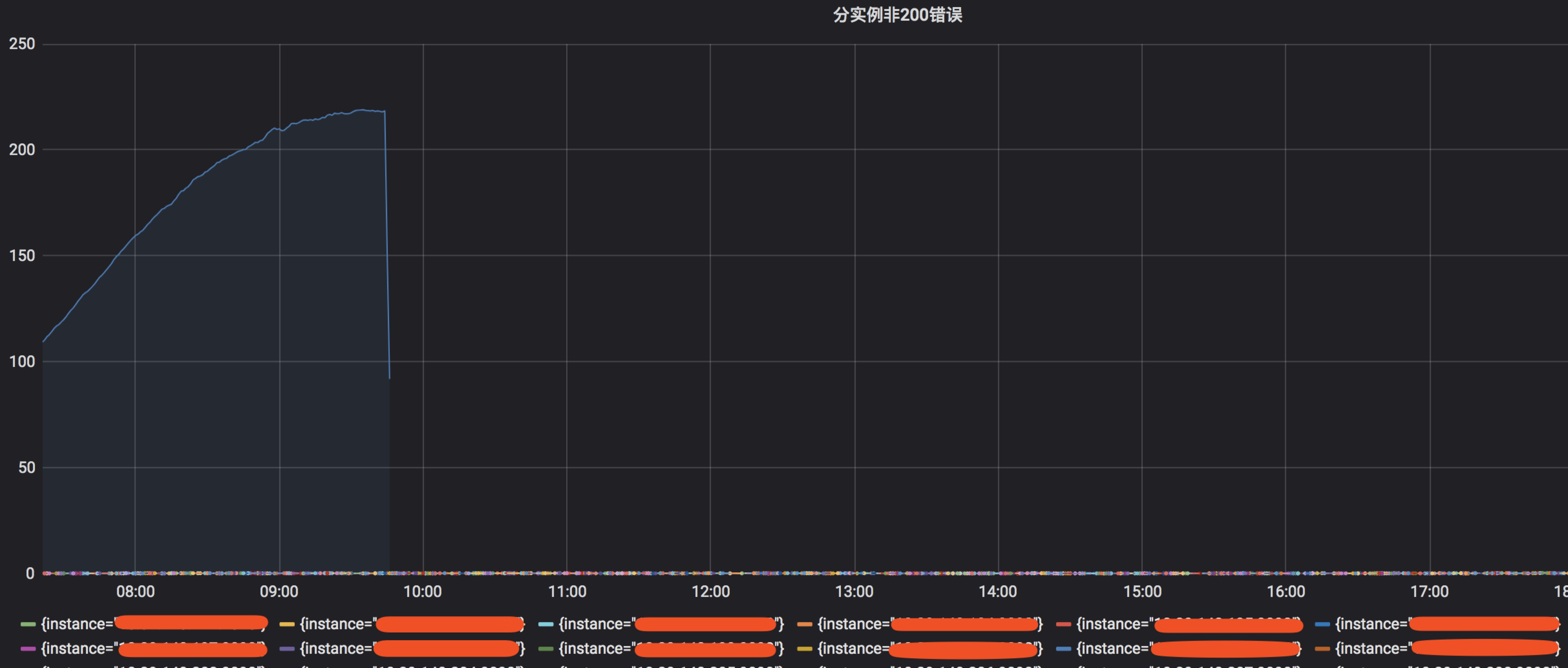# 玩转Prometheus(4)–发现异常节点

版权声明 本站原创文章 由 萌叔 发表



### 2. 分析&展示报警

• 请求超时
• 请求错误多

#### 2.1 监控图表

topk(3, sum(rate(http_requests_total{project="fake-service", run_mode="product", status!~"200|201|204"}[5m])) by (instance))#### 2.2 配置报警

某个实例指标的值 > 所有实例指标的平均值 + 2 * 所有实例指标的标准差


DSL

floor(max(sum(rate(http_requests_total{project="sdk-api", run_mode="product", status!~"200|201|204"}[5m])) by (instance))) >  avg(sum(rate(http_requests_total{project="sdk-api", run_mode="product", status!~"200|201|204"}[5m])) by (instance))  +  2 * stddev(sum(rate(http_requests_total{project="sdk-api", run_mode="product", status!~"200|201|204"}[5m])) by (instance))


### 参考资料

#### 如果我的文章对你有帮助，你可以给我打赏以促使我拿出更多的时间和精力来分享我的经验和思考总结。